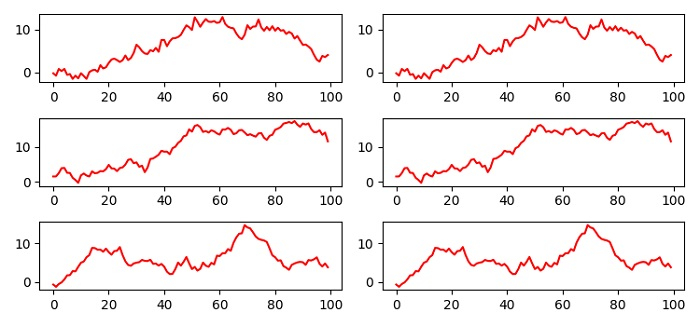# Populating Matplotlib subplots through a loop and a function

MatplotlibPythonData Visualization

To populate matplotlib subplots through a loop and a function, we can take the following steps −

• Set the figure size and adjust the padding between and around the subplots.

• Create a figure and a set of subplots with number of rows = 3 and number of columns = 2.

• Make a function to iterate the columns of each row and plot the x data points using plot() method at each column index.

• Iterate rows (Step 2) and create random x data points and call iterate_columns() function (Step 3).

• To display the figure, use show() method.

## Example

import numpy as np
import matplotlib.pyplot as plt

plt.rcParams["figure.figsize"] = [7.50, 3.50]
plt.rcParams["figure.autolayout"] = True

fig, axes = plt.subplots(3, 2)

""" Iterate column's axes"""
def iterate_columns(cols, x):
for col in cols:
col.plot(x, color='red')

""" Iterate row's axes"""
for row in axes:
x = np.random.normal(0, 1, 100).cumsum()
iterate_columns(row, x)

plt.show()

## Output